# shovelingpig / Super_Resolution_with_CNNs_and_GANs

Image Super-Resolution Using SRCNN, DRRN, SRGAN, CGAN in Pytorch

Geek Repo

Github PK Tool

# Super Resolution with CNNs and GANs

This is the code for our cs231n project.

We investigated the problem of image super-resolution (SR), where we want to reconstruct high-resolution images from low-resolution images. We presented a residual learning framework to ease the training of the substantially deep network. Specifically, we reformulated the structure of the deep-recursive neural network to improve its performance. To further improve image qualities, we built a super-resolution generative adversarial network (SRGAN) framework, where we proposed several loss functions based on perceptual loss, i.e. SSIM loss and/ or total variation (TV) loss, to enhance the structural integrity of generative images. Moreover, a condition is injected to resolve the problem of partial information loss associated with GANs.

The results show that our methods and trails can achieve equivalent performance on most of the benchmarks compared with the previous state-of-art methods, and out-perform them in terms of the structural similarity. Here are a few example outputs: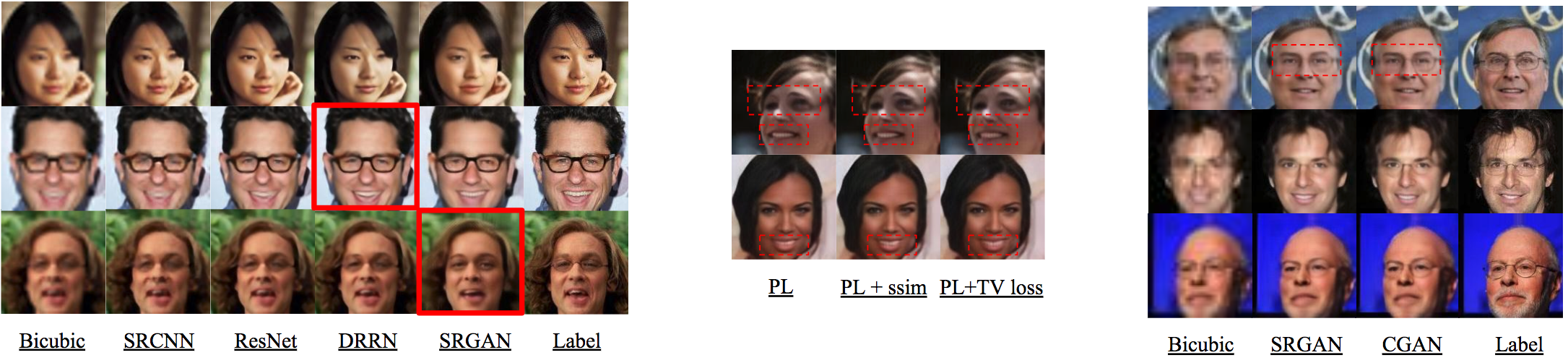If you find this code useful in your project, please star this repository and cite:

``````@inproceedings{densecap,
title={Super Resolution with CNNs and GANs},
author={Yiyang, Li and Yilun, Xu and Ji, Yu},
year=2018,
}
``````

## Installation

This project was implemented in PyTorch 0.4 and Python3

## Quickstart

1. Build datasets: First, you need to download the dataset. We use CelebA as our dataset. e.g for cnn_based model, we set input size 144×144 and output size 144×144; For gan model, we set input size 36×36 and output size 144×144
``````python build_dataset.py --data_dir ../img_align_celeba_test --output_dir ../data/cnn_faces --input_size 144 --output_size 144
``````
1. Train your experiment

for cnn-based models, e.g SRCNN:

``````python train_cnn.py --data_dir ../data/cnn_faces --model_dir experiments/srcnn_model --model srcnn --cuda cuda0 --optim adam
``````

for gan models, e.g SRGAN:

``````python train_gan.py --data_dir ../data/gan_faces --model_dir experiments/gan_model --model gan --cuda cuda0 --optim adam
``````

If you want to train the model from last time, add this:

``````--restore_file "best"
``````
1. Perform hyperparameters search e.g srcnn from cnn_based model
``````python search_hyperparams.py --data_dir ../data/cnn_faces --parent_dir experiments/learning_rate --model srcnn --model_type cnn
``````
1. Display the results of the hyperparameters search in a nice format
``````python synthesize_results.py --parent_dir experiments/learning_rate
``````
1. Evaluation on the test set

for cnn-based models, e.g SRCNN:

``````python evaluate_cnn.py --data_dir ../data/cnn_faces --model_dir experiments/srcnn_model --model srcnn --cuda cuda0
``````

for gan models, e.g SRGAN:

``````python evaluate_gan.py --data_dir ../data/gan_faces --model_dir experiments/gan_model --model gan --cuda cuda0
``````

## Result List

1. SRCNN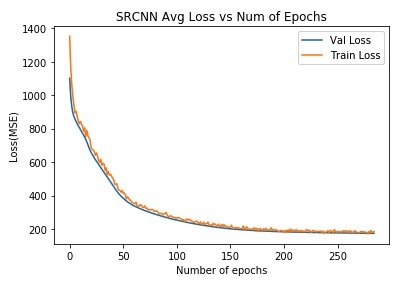1. DRRN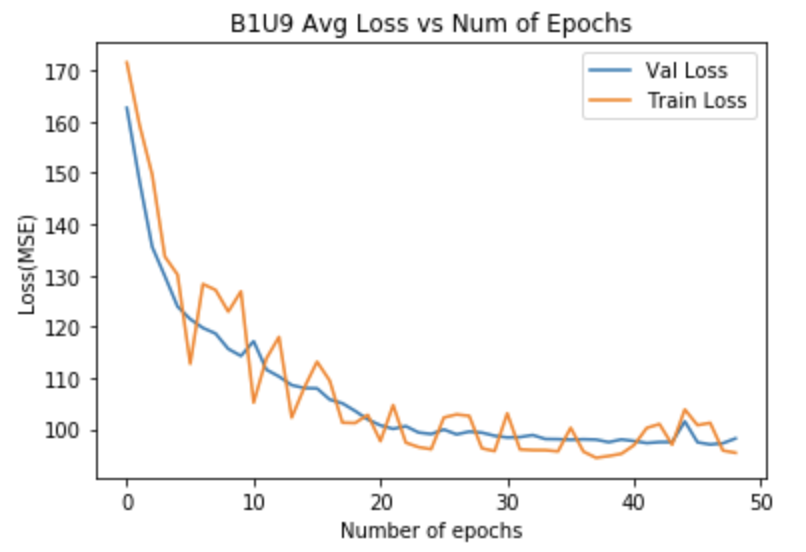1. SRGAN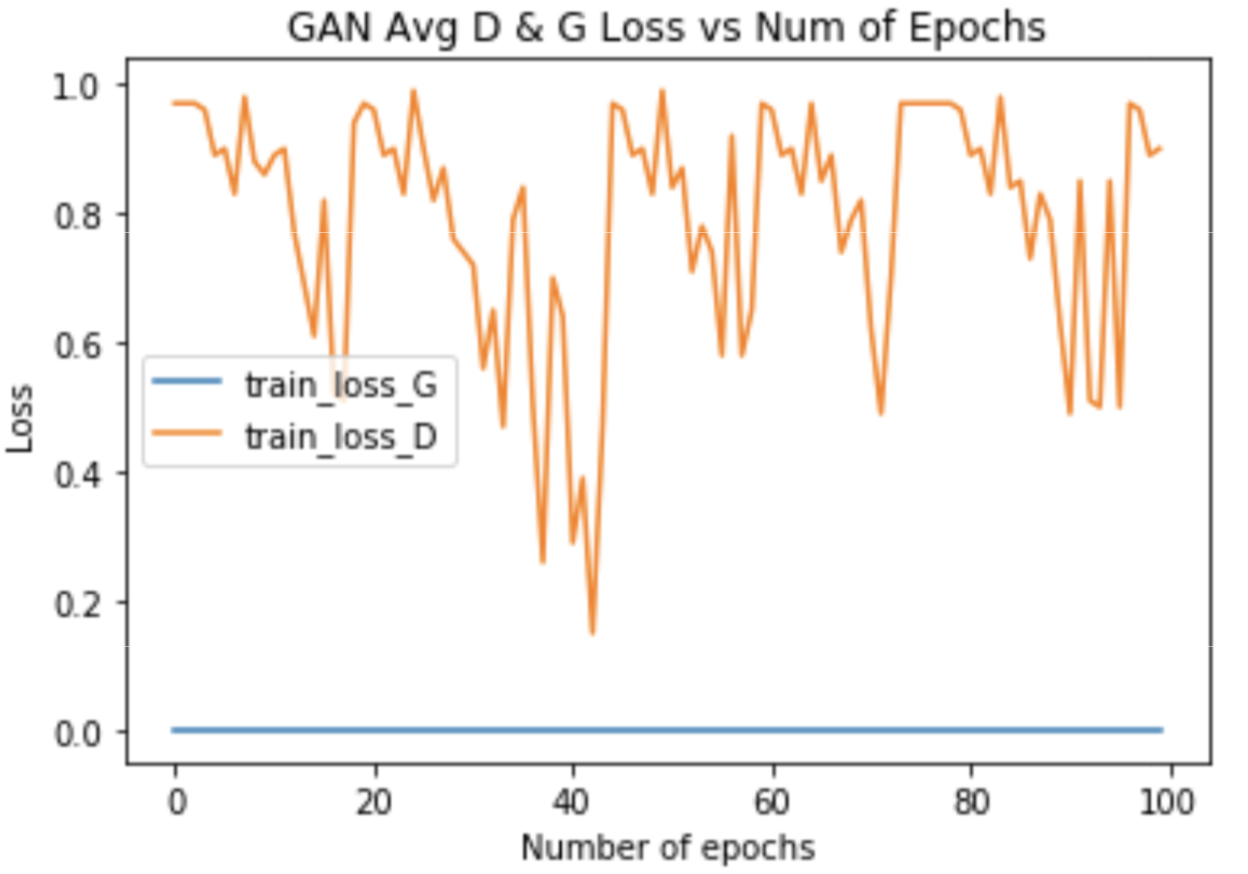1. CGAN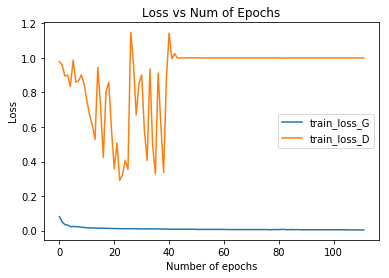## Pre-trained Models

The following Pytorch models were trained on CelebA dataset:

1. SRCNN(best.pth.tar)
2. DRRN(best.pth.tar)
3. SRGAN(best.pth.tar)
4. CGAN(best.pth.tar)

## Reference

 CS230 Stanford, https://github.com/cs230-stanford/, cs230-code-examples, 2018.

 tyshiwo, Drrn CVPR17, https://github.com/tyshiwo/DRRN_CVPR17, 2017.

 leftthomas, Srgan, https://github.com/leftthomas/SRGAN, 2017.

 znxlwm, pytorch-generative-modelcollections, https://github.com/znxlwm/pytorch-generative-model-collections, 2017.